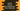# Python remove the last inserted item from a dictionary using popitem()## Python dictionary popitem() method:

Python dictionary popitem method can be used to remove the last inserted item to an dictionary in Python. Before python 3.7, this method removes one random value from a dictionary. Starting from python 3.7, we can use it to remove the last inserted value to an dictionary.

In this post, we will learn how to use Python popitem method with examples.

## Definition:

This method is defined as below:

``dict.popitem()``

Here, dict is the calling dictionary.

The return value of this method is a tuple holding the removed values from the dictionary. If the dictionary is empty, i.e. if it can’t find any value to remove, it throws an error.

Let’s check how this method works with different examples.

### Example 1: Remove the last inserted item from a dictionary:

Let’s take a look at the below program:

``````given_dict = {'one': 1, 'two': 2}

given_dict['three'] = 3

print('given_dict : {}'.format(given_dict))

print(given_dict.popitem())

print('given_dict : {}'.format(given_dict))``````

Here,

• given_dict is the given dictionary. It has two key-value pairs.
• Then we are adding one more key-value pairs to the dictionary.
• The first print statement prints the dictionary values before calling popitem on it.
• The second print statement prints the value that popitem returns.
• The final print statement again prints the dictionary values.

If you run this program, it will print the below output:

``````given_dict : {'one': 1, 'two': 2, 'three': 3}
('three', 3)
given_dict : {'one': 1, 'two': 2}``````

As you can see, popitem returns the last item inserted to the dictionary.

### Example 2: Calling popitem on an empty dictionary:

Let’s try to call popitem on an empty dictionary:

``````given_dict = {'one': 1, 'two': 2}

print('given_dict : {}'.format(given_dict))

print(given_dict.popitem())
print(given_dict.popitem())
print(given_dict.popitem())``````

given_dict has two values. But we are calling popitem three times. The first two popitem methods will work, but the third one will throw one error.

``KeyError: 'popitem(): dictionary is empty'``